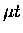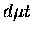Next: Implications for our measurements Up: Back-decayed energy distribution Previous: Resonant excitation of DX

## Back-decayedenergy

As we have seen, the energy distribution of back-decayeddepends on the details of back decay reactions as well as MMC interactions with the surrounding environment, which include: (a) the back decay matrix elements, (b) collisional ro-vibrational relaxation of the MMC, (c) the MMC elastic scattering cross section for , and perhaps (d) solid state effects such as emission of phonons upon back decay and recoilless MMC decay. Apart from the plausible yet still speculative effect (d), the processes (a) and (b) affect the Q-value of the back decay reaction, while (c) changes the motion of the MMC centre of mass frame, hence affecting theenergy in the lab frame.

The complete analysis of this complex chain of processes is beyond the scope of this appendix, and we deal with two limiting cases of (b), i.e., no rotational relaxation at all, and complete rotational relaxation (no vibrational relaxation assumed in either case). We develop in the following expressions for the double differential rates for resonant scattering which depend on both outgoing and incomingenergies in the lab frame . Our formulation is analogous to the resonant formation rate calculations by Faifman et al. . We explicitly treat the back-decayedenergy , paying attention to the energy balance. Thermal motion of the MMC is included to give the Doppler broadening  of the distribution.

In the first limit of no relaxation at all in the MMC states, the rate for the resonant scattering rate can be written:

 = (137)

where

 (138)

is theenergy from the decay channel with

 (139)

being the binding energy difference between the initial and final state of DX (i.e., excitation or de-excitation energy of DX due to the resonant scattering). Other notations used in Eq. B.13 include:
• (As usual) S,F denote the spin ofand hyperfine state of, respectively.
• is the resonance intensity profile for detuning (e.g., in Faifman's model).
• D(Eu) is the Doppler broadening distribution due to the motion of MMC at the time of back decay (e.g., Gaussian distribution for thermalized MMC if ).
• is the back decay width for the channel with given S,F.
• is the total formation rate for the incidentlab energy .
• is the initial distribution of target molecules DX.

In the other limit that MMC rotational relaxation is complete, the following substitution should occur:

 (140) (141)

with being the Boltzmann distribution for the rotational states of MMC, hence simplifying the summation in Eq. B.13.

Because the expression given in Eq. B.13 is rather complicated, we can alternatively take advantage of the already calculated formation ratesand fusion probability WF to write, in the limit of no MMC rotational relaxation, that(142)

where
 = (143)

is the energy distribution of back-decayedfor a given initial energy , and

 (144)

is the branching ratio for decay to the state , given the MMC state of , and

 (145)

is the conditional probability that the MMC has the state , given that the MMC is formed with aof energy . Again, in the other limit of complete MMC rotational relaxation, we can average Kf states over the Boltzmann distribution, and let

together with the substitution in Eq. B.17.

Note in Eq. B.18, we made an approximation by factorizing the state dependence, and this is not rigorously accurate when the state dependence of each factor is large, hence the approach given here should be taken as a first approximation. On the other hand, the first expression given in Eq. B.13 does not rely on the factorization approximation hence it is more accurate, though more complicated.

In order to numerically evaluate these expressions, we need some several hundred matrix elements for MMC transitions. It should be stressed, however, these have been already calculated, for example by Faifman et al. for calculations of, thus with their assistance we can readily estimate the energy distribution of back-decayed(within the approximation that the MMC is translationally thermalized, and is rotationally relaxed or not relaxed at all).

We note that in case of the elastic scattering, with translationally thermalized MMC, the energy of back-decayed is given by the resonance energy divided betweenand DX (convoluted with the Doppler broadening profile). Hence the claims by Somov and Jeitler [238,74,22] of thermalizedafter back decay is not physically justified. Jeitler also considered the limit of no MMC rotational relaxation at all, but the simple expression given for theenergy distribution for that case ( , Eq. 4.72 in Ref. ) is not accurate, as the back decay into (the resonant excitation of DX), and the Doppler broadening due to MMC thermal motion are neglected.Next: Implications for our measurements Up: Back-decayed energy distribution Previous: Resonant excitation of DX